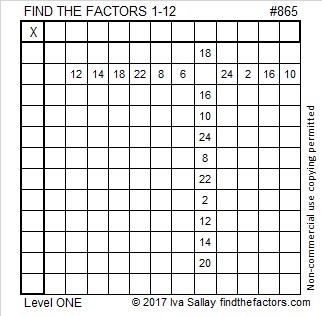# 865 and Level 1Print the puzzles or type the solution on this excel file: 12 factors 864-874

865 is the sum of two squares two different ways:

• 28² + 9² = 865
• 24² + 17² = 865

865 is the hypotenuse of four Pythagorean triples, two of which are primitives:

• 260-825-865, which is 5 times (52-165-173)
• 287-816-865, which is 24² – 17², 2(24)(17), 24² + 17²
• 504-703-865 which is 2(28)(9), 28² – 9², 28² – 9²
• 519-692-865, which is (3-4-5) times 173

You could see 865’s factors in two of those Pythagorean triples, and here they are again:

• 865 is a composite number.
• Prime factorization: 865 = 5 × 173
• The exponents in the prime factorization are 1 and 1. Adding one to each and multiplying we get (1 + 1)(1 + 1) = 2 × 2 = 4. Therefore 865 has exactly 4 factors.
• Factors of 865: 1, 5, 173, 865
• Factor pairs: 865 = 1 × 865 or 5 × 173
• 865 has no square factors that allow its square root to be simplified. √865 ≈ 29.41088

## 2 thoughts on “865 and Level 1”

1.davescarthin

I like the way that although 865 does not have an integral square root it relates two pairs of integers, (28,9) and (24,17), by being the common hypotenuse of two right-angled triangles. The Pythagorean case when one of the four numbers thus related is zero collapses one of the triangles into just a line – much less beautiful?

•ivasallay

I’m not sure what you meant when you wrote, “The Pythagorean case when one of the four numbers thus related is zero collapses one of the triangles into just a line – much less beautiful?” Are you saying that 865² + 0² = 865² is not as beautiful as 287² + 816² = 865²?

This site uses Akismet to reduce spam. Learn how your comment data is processed.